## PKL Electrochemical Cell -A New and Innovative Clean Energy Production System

Md. Kamrul Alam Khan1*

1Department of Physics, Jagannath University, Dhaka-1100, Bangladesh.

*Corresponding Author: Md. Kamrul Alam Khan, Department of Physics, Jagannath University, Dhaka-1100, Bangladesh, Tel: +8801911357447; Fax: +8801911357447; E-mail: kakhan01@yahoo.com

Citation: Md. Kamrul Alam Khan (2020) PKL Electrochemical Cell -A New and Innovative Clean Energy Production System. Nano Technol & Nano Sci J 3: 118.

Copyright: © 2020 Md. Kamrul Alam Khan, et al. This is an open-access article distributed under the terms of the Creative Commons Attribution License, which permits unrestricted use, distribution, and reproduction in any medium, provided the original author and source are credited.

Received: July 04, 2020; Accepted: July 13, 2020; Published: July 16, 2020.

## Abstract

In this research work it has been conducted the performances of PKL electricity for practical utilization in DC appliances. The parameters like open circuit voltage (Voc), Short circuit current (Isc), discharge voltage (Vd), discharge current (Id), power, current density, power density, energy efficiency, columbic efficiency voltage efficiency have been developed. The parameters have been measured by sophisticated multimeters with different DC appliances as a load. The variation of open circuit voltage (Voc), Short circuit current (Isc), discharge voltage (Vd), discharge current (Id), power, current density, power density, energy efficiency, columbic efficiency voltage efficiency with the variation of different day of the weeks have been studied. It is found that the performances decreased firstly and then increased. Then it can be concluded that the PKL electrochemical cell has retarding force. The observations have been taken for different weeks. The findings of this article are that the PKL electrochemical cell is feasible and viable for DC appliances for practical utilizations. It was found that the maximum energy efficiency was 38.38% and the minimum energy efficiency was 25.85%. It was also found that the maximum columbic efficiency was 92.43% and the minimum columbic efficiency was 62.63%. It was also found that the maximum voltage efficiency was 41.98% and the minimum voltage efficiency was 41.51% for 672.5 hrs. Furthermore, the maximum open circuit voltage (Voc) was 5.18volt, Short circuit current (Isc) was 2.87 mA, power was 14.87 mW, current density was 0.052mA/cm2 and the minimum open circuit voltage (Voc) was 5.105 volt, Short circuit current (Isc) was 2.85 mA, power was 14.71 mW, current density was 0.051 mA/cm2for 672.5 hrs.

## Keywords

Power, Current Density; Energy Efficiency; Columbic Efficiency; Voltage Efficiency.

## Introduction

The traditional sources of energy like oil, gas and coal are the mineral sources . These are limited source. Because it will be finished within 2100 centuries. So we need to conduct research on new and renewable energy sources . The energy source which does not have a limited source and which can be generated again and again and will never run out is called renewable energy sources. These are solar energy, Bio gas energy, Bio mass energy, Geo thermal energy, Tidal energy, Hydro power, wave energy and OTEC (Ocean Thermal Energy Conversion) etc. The solar energy is very popular now a day in Bangladesh . The solar energy is divided in different ways like solar home system (SHS), solar building integrated system, solar grid connected system and solar floating system etc. The solar home system (SHS) mainly depends on DC appliances [4,5]. There are some problems for using solar home system (SHS). It needs storage battery during night time. But the storage battery does not stay long time. So that after some years it needs to replace by a new storage battery. That is why people are not happy on storage battery [6,7]. They have to purchase new battery to run the solar home system smoothly. To keep it in mind we have designed and developed our PKL electric system for DC appliances [8,9]. We studied several parameters for use in DC appliances. Most of the results have been tabulated and graphically discussed.

## Objectives

(i)To disseminate PKL electricity for DC appliances.

(ii) To grow interest for PKL electricity among the people.

## Methodology

The different Specifications of Electrolyte is given below:

Quantity of PKL = 800g = 0.8kg, Quantity of water = 3200ml = 3.2liter and PKL: Water = 1:4 and the dimensions of the Zn electrode islength of the Zn electrode = 14.8cm, width of the Zn Electrode = 3.8cm and the area of the Zn electrode = (14.8x3.8) cm2 = 56.24cm2. The dimensions of each Chamber is Length of each Chamber = 10.0cm,Width of each Chamber = 3.5cmand thearea of each Chamber = (10.0x3.5) cm2 = 35cm2.

Figure 1: Experimental set up for the DC appliances of PKL electricity.

Figure 1(a): PKL electricity production components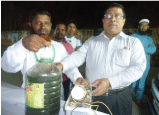Figure 1(b): DC stand fan with PKL electricityFigure 1(c): DC LED light with PKL electricity.It is shown in Figure 1(a) that the PKL extract & DC LED bulb with PKL electricity, in Figure 1(b) about the DC table fan PKL with PKL electricity and in Figure 1(c) regarding the DC bulb with PKL electricity. Overall Figure 1 shows the experimental set up for the DC appliances of PKL electricity.

Electrodes: The zinc plate was used as an anode and the copper plate was used as a cathode. There are 6 chambers/compartments in a PKL module, which was made by an insulated battery box . There are one pair of electrodes (one Zinc plate and one copper plate) are set up in each compartment. Then electrodes of each compartment are connected in series combination. As a result the voltage of the PKL module increases and the current of the PKL module remain constant. Then after the DC load is connected between the ends of the PKL module. If load needs high voltage then more PKL module is connected in series connection to face the demand of the DC load voltage. The electrolyte prepared by PKL extract has been put in to the each compartment of the PKL module. After a chemical reaction the voltage is generated in the PKL module which lights the DC appliances.

Electrolytes: PKL extract mixed with water for getting electrolyte of the PKL electrochemical cell. This PKL extract has been filtered and thenkeep itinto the each compartment of the PKL electrochemical Cell. When the electrodes Zinc and copper are immersed in to the electrolyte then the voltage and current are produced which lights the DC appliances [11-12].

Battery box: The battery box was made by an insulated material like plastics. Generally, it is available in the local market.

Connecting wires: The connecting wires are made of copper. It is also available in the market. It can be purchased from both local and International market. Although it is also available in the local market. It can be purchased by kg or, feet basis.

Multimeters: The voltage and current have been measured by a calibrated multimeters. It can be purchased from both local and International market. Although it is also available in the local market.

PKL cultivation: The PKL is called miracle leaf. Because it can grow everywhere. So that it can be harnessed easily. After collecting the PKL it has been blended by a blender or any other suitable crash machine. Then it is mixed with water. If the water is DI water then it would better for better performance of electricity generation.

## Chemical Reactions

The difference between cathode and anode for a PKL electrochemical cell is given below which is shown in Table-1.

Table 1: Difference between cathode and anode for a PKL electrochemical cell.

 Copper electrode as a cathode Zinc electrode as an anode Where gain of electrons Where loss of electrons Where reduction occurs Where oxidation occurs It has positive(+)sign It has negative(-)sign Where + ve ions migrate to copper Where – ve ions migrate to zinc

Generation of Electric potential in a PKL System: The potential difference between Cu plate and Zn plate of a PKL cell is called electric potential or electromotive force of a PKL electrochemical cell. Generally, it is denoted by Ecell. It is measured by the equation, 1V= 1J/1C (since, V= W/Q) …….. ………………… …. … (1),

Where, V = potential, W= Potential Energy and Q= charge. The pull or driving force on electrons of a PKL electrochemical cell is also called the electromotive force (e.m.f). The causes of the electro motion in a PKL electrochemical cell is the electromotive force. The potential at Zn plate may be called the oxidation potential and the potential at Cu plate may be called the reduction potential [13,14]. The electrode potential cannot be measured in isolation. The electrode potential of a PKL cell depends upon the chemical properties of the materials of the electrodes, concentration of the materials, the temperature and pressure [15,16].

Standard State Condition for PKL electrochemical cell: Concentration of the solution = 1 mol/L, The pressure of gases = 1 atm. and Temperature = 25ºC or 298 K.

Standard PKL electrochemical Cell Potentials: The PKL electrochemical cell potential at standard state conditions can be shown by the following equation:

cell = Eºred (cathode) − Eºred (anode) …….. …… …… ….. (2),

where, Eºcell = Cell potential, Eºred (cathode) = Cathode (Cu plate) potential or, reduction potential and Eºred (anode) = Anode (Zn plate) potential or oxidation potential. The intensive property of the PKL electrochemical cell can be found according to equation (1), The potential energy per unit of charge is equal to the potential of the PKL electrochemical cell. For PKL electrochemical cell, Eºred (cathode) = -0.76 volt and Eºred (anode) = +0.34 volt. Therefore, from equation (2), we have, Eºcell= +0.34-(-0.76)=1.10 volt . The strongest oxidizers have the most +ve reduction potential and the strongest reducers have the most –ve oxidation potential. It is found that the PKL cell potential depends upon the difference of the potential of two electrodes. It is also shown that the reduction potential of the PKL cell potential does not depend upon the stoichiometric constant.

Summary of the standard electrode potential: The electric voltage is created of a PKL electrochemical cell for two dissimilar electrode materials. This voltage has been measured for the potential energy per unit charge from the redox reaction to drive the total reaction activities. The PKL electrochemical cell has two half cells made by cathode (Cu plate) and anode (Zn plate) with PKL extract. The important and interesting matter is that the created voltage of the PKL electrochemical cell is positive because the reaction is spontaneous [18,19].

Difference between Cathodic and Anodic reactions of a PKL electrochemical Cell:

Table 2: Difference between cathodic and anodic reactions for a PKL electrochemical cell.

 Cathodic Reactions Anodic Reactions Cu2+ + 2e- = Cu Cu-2e- = Cu2+ Zn2+ + 2e- = Zn Zn-2e- = Zn2+ Fe3++e- = Fe2+ Fe2+ - e- = Fe3+ H+ +2e- = 2H= H2 2H-e- = H+

It is shown in Table 2 that the Zn2+ is the product ion and the Cu2+, Zn2+ and the H+ are the reactant ions. In PKL electrochemical system, the concentration of the reactant ions should be higher than the concentration of the product ions.

## Results and Discussion

To study the performance of PKL electrochemical cell some parameters have been studied and tabulated in Table-3. The different parameters have been collected for the same experimental setup after a week. It is mentioned that the specifications for Table 3 have been taken after 5 weeks from starting date of the PKL electrochemical cell.

Table 3: Data Collection for different parameters.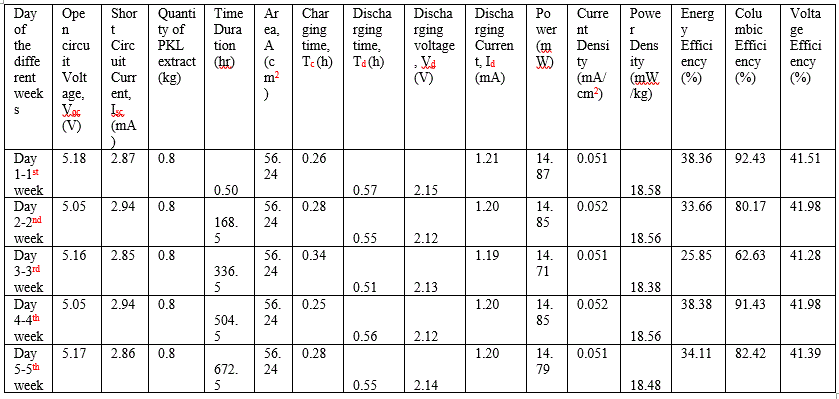Figure 2: Voc – Time duration (hr).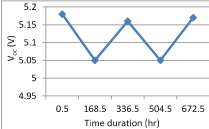Figure 3: Isc – Time duration (hr).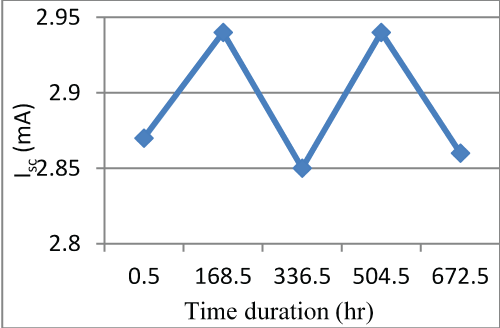Figure 4: Vd – Time duration (hr).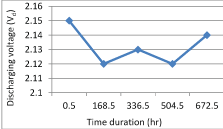Figure 2 shows that the open circuit voltage VOC decreases from 5.18 volt (at 0.5 hr) to 5.05 volt at 168.5 hr linearly and then it increases from 5.05 volt to 5.16 volt at 336.5 hr linearly. Then after it decreases from 5.16 volt to 5.05 volt at 504.5 hr linearly. Finally, it increases from 5.05 volt to 5.17 volt (5th week) at 672.5 hr linearly. It is concluded from the above analysis that the reading for data collection was taken after a week. The PKL electrochemical cell regained electric energy and then discharge during data collection. It is found that the difference between lowest and highest voltage is 0.13 volt for 5 weeks.

Figure 3 shows that the short circuit current Isc increases from 2.87 mA (1st week) to 2.94 mA (2nd week) at 168.5 hr linearly and then it decreases from 2.94 mA (2nd week) to 2.85 mA (3rd week) at 336.5 hr linearly. Then after it increases from 2.85 mA (3rd week) to 2.94 mA (4th week) at 504.5 hr linearly. Finally, it decreases from 2.94 mA (4th week) to 2.86mA (5th week) at 672.5 hr linearly. It is concluded from the above analysis that the reading for data collection was taken after a week. The PKL electrochemical cell regained electric energy and then discharge during data collection. It is found that the difference between lowest and highest current is o.09 mA for 5 weeks.

Figure 4 shows that the load voltage Vd decreases from 2.15 volt (1st week) to 2.12 volt (2nd week) at 168.5 hr linearly and then it increases slightly from 2.12 volt (2nd week) to 2.13 volt (3rd week) at 336.5 hr linearly. Then after it decreases from 2.13 volt (3rd week) to 2.14 volt (4th week) at 504.5 hr linearly. Finally, it increases from 2.12 volt (4th week) to 2.14 volt (5th week) at 672.5 hr linearly. It is concluded from the above analysis that the reading for data collection was taken after a week. The PKL electrochemical cell regained electric energy and then discharge during data collection. It is found that the difference between lowest and highest voltage is 0.03 volt for 5 weeks.

Figure 5: Id – Time duration (hr).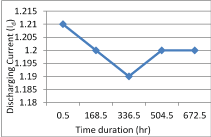Figure 6: Power – Time duration (hr).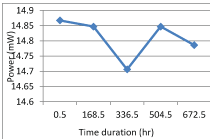Figure 7: Current density – Time duration (hr).Figure 5 shows that the discharge current Id decreases from 1.21 mA (1st week) to 1.20 mA (2nd week) at 168.5 hr linearly and then it decreases from 1.20 mA (2nd week) to 1.19 mA (3rd week) at 336.5 hr linearly. Then after it increases from 1.19 mA (3rd week) to 1.20 mA (4th week) at 504.5 hr linearly. Finally, it was steady from 1.20 mA (4th week) to 1.20 mA (5th week) at 672.5 hr which was completely linear. It is concluded from the above analysis that the reading for data collection was taken after a week. The PKL electrochemical cell regained electric energy and then discharge during data collection. It is found that the difference between lowest and highest current is o.02 mA for 5 weeks.

Fig-6 shows that the power decreases from 14.87 mW (1st week) to 14.85 (2nd week) at 168.5 hr linearly and then it increases slightly from 14.85 mW (2nd week) to 14.71 mA (3rd week) at 336.5 hr linearly. Then after it decreases from 14.71 mA (3rd week) to 14.85 mA (4th week) at 504.5 hr linearly. Finally, it increases from 14.85 mA (4th week) to 14.79 mA (5th week) at 672.5 hr linearly. It is concluded from the above analysis that the reading for data collection was taken after a week. The PKL electrochemical cell regained electric power during data collection. It is found that the difference between lowest and highest voltage is 0.16 mA for 5 weeks.

Figure 7 shows that the current density increases from 0.051 mA/cm2 (1st week) to 0.052 mA/cm2 (2nd week) at 168.5 hr linearly and then it decreases from 0.051 mA/cm2 (2nd week) to 0.051 mA/cm2 (3rd week) at 336.5 hr linearly. Then after it increases from 0.051 mA/cm2to 0.052 mA/cm2 (4th week) at 504.5 hr linearly. Finally, it decreases from 0.052 mA/cm2 (4th week) to 0.051 mA/cm2 (5th week) at 672.5 hr linearly. It is concluded from the above analysis that the reading for data collection was taken after a week. The PKL electrochemical cell regained electric energy and then discharge during data collection. It is found that the difference between lowest and highest current is 0.001 mA/cm2 for 5 weeks.

Figure 8: Power density – Time duration (hr).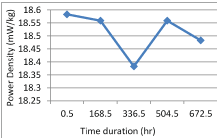Figure 9: Energy efficiency – Time duration (hr).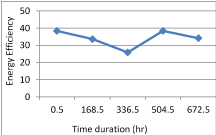Figure 10: Columbic efficiency – Time duration (hr).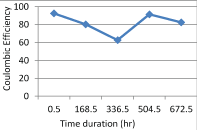Figure 8 shows that the power density decreases from 18.58 mW/kg (1stweek) to 18.56 mW/kg (2nd week) at 168.5 hr linearly and then it decreases slightly from 18.56 mW/kg (2nd week) to 18.38 mW/kg (3rd week) at 336.5 hr linearly. Then after it increases from 18.38 mW/kg (3rd week) to 18.56 mW/kg (4th week) at 504.5 hr linearly. Finally, it decreases from 18.56 mW/kg (4th week) to 18.48 mW/kg (5th week) at 672.5 hr linearly. It is concluded from the above analysis that the reading for data collection was taken after a week. The PKL electrochemical cell regained electric power during data collection. It is found that the difference between lowest and highest voltage is 0.20 mW/kg for 5 weeks.

Figure 9 shows that the energy efficiency (%) decreases from 38.36(%) (1st week) to 33.66(%) (2nd week) at 168.5 hr linearly and then it decreases slightly from 33.66(%) (2nd week) to 25.85(%) (3rd week) at 336.5 hr linearly. Then after it increases from 25.85(%) (3rd week) to 38.38(%) (4th week) at 504.5 hr linearly. Finally, it decreases from 38.38(%) (4th week) to 34.11(%) (5th week) at 672.5 hr linearly. It is concluded from the above analysis that the reading for data collection was taken after a week. The PKL electrochemical cell regained electric power during data collection. It is found that the difference between lowest and highest voltage is 12.53(%) for 5 weeks.

Figure 10 shows that the columbic efficiency (%) decreases from 92.43(%) (1st week) to 80.17(%) (2nd week) at 168.5 hr linearly and then it decreases slightly from 80.17(%) (2nd week) to 62.63(%) (3rd week) at 336.5 hr linearly. Then after it increases from 62.63 (%) (3rd week) to 91.43 (%) (4th week) at 504.5 hr linearly. Finally, it decreases from 91.43 (%) (4th week) to 82.42 (%) (5th week) at 672.5 hr linearly. It is concluded from the above analysis that the reading for data collection was taken after a week. The PKL electrochemical cell regained electric power during data collection. It is found that the difference between lowest and highest voltage is 29.80(%) for 5 weeks.

Figure 11: Voltage efficiency - Time duration (hr) curve.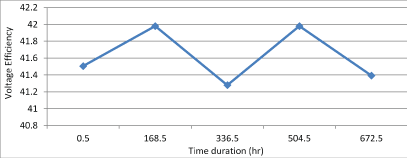Figure 11 shows that the voltage efficiency (%) increases from 41.51(%) (1st week) to 41.98(%) (2nd week) at 168.5 hr linearly and then it decreases slightly from 41.98(%) (2nd week) to 41.28(%) (3rd week) at 336.5 hr linearly. Then after it increases from 41.28 (%) (3rd week) to 41.98 (%) (4th week) at 504.5 hr linearly. Finally, it decreases from 41.98 (%) (4th week) to 41.39 (%) (5thweek) at 672.5 hr linearly. It is concluded from the above analysis that the reading for data collection was taken after a week. The PKL electrochemical cell regained electric power during data collection. It is found that the difference between lowest and highest voltage is 0.70 (%) for 5 weeks.

## Conclusion

The stronger oxidizing agent and the weaker reducing agent make a positive electro potential for PKL electrochemical system. It is in oxidized form for more negative reduction potential which makes the weaker oxidizing agent and it is reduced form for the stronger reducing agent. The positive value of Cell potential indicates the overall reaction is spontaneous. The electricity is produced due to the voltage difference between two Zn and Cu electrodes for PKL electrochemical cell. This voltage difference is also the difference between two individuals voltage of Zn and Cu electrodes with respect to the PKL extract electrolyte. The overall voltage of PKL electrochemical cell can be measured properly but there is no simple way to properly measure the voltage between electrode-electrolyte till today. The electric voltage of the PKL electrochemical cell depends upon concentration, temperature and pressure.

## Acknowledgement

The authors are grateful to the GARE (Grant of Advanced Research in Education) project, Ministry of Education, GoB for financing during the research work (Project/User ID: PS2019949).